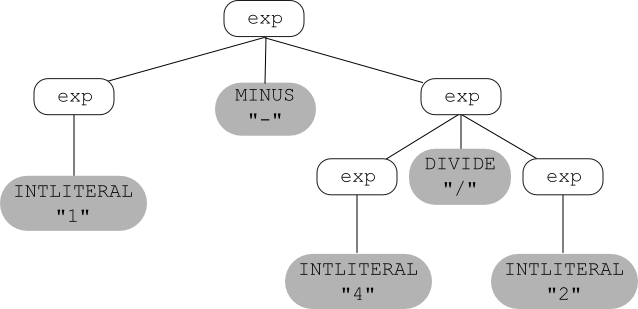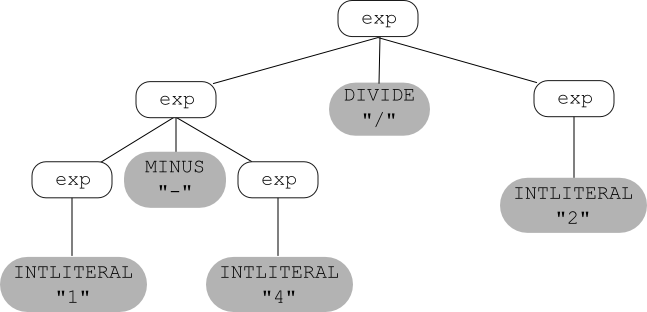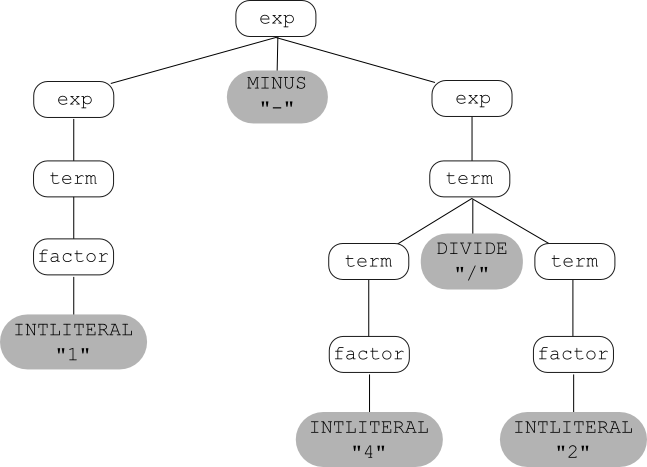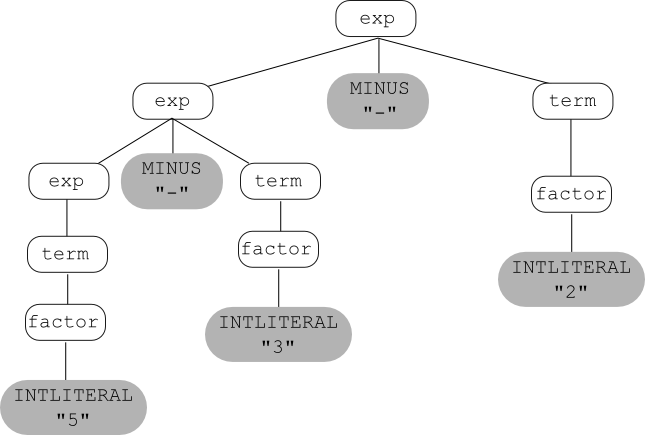Contents Overview

Recall that the input to the parser is a sequence of tokens (received interactively, via calls to the scanner). The parser:

• Groups the tokens into "grammatical phrases".
• Discovers the underlying structure of the program.
• Finds syntax errors.
• Perhaps also performs some actions to find other kinds of errors.
The output depends on whether the input is a syntactically legal program; if so, then the output is some representation of the program:
• an abstract-syntax tree (maybe + a symbol table),
• or intermediate code,
• or object code.
We know that we can use regular expressions to define languages (for example, the languages of the tokens to be recognized by the scanner). Can we use them to define the language to be recognized by the parser? Unfortunately, the answer is no. Regular expressions are not powerful enough to define many aspects of a programming language's syntax. For example, a regular expression cannot be used to specify that the parentheses in an expression must be balanced, or that every else'' statement has a corresponding if''. Furthermore, a regular expression doesn't say anything about underlying structure. For example, the following regular expression defines integer arithmetic involving addition, subtraction, multiplication, and division:
digit+ (("+" | "-" | "*" | "/") digit+)*

but provides no information about the precedence and associativity of the operators.

So to specify the syntax of a programming language, we use a different formalism, called context-free grammars.

We can write a context-free grammar (CFG) for the language of (very simple) arithmetic expressions involving only subtraction and division. In English:

• An integer is an arithmetic expression.
• If exp1 and exp2 are arithmetic expressions, then so are the following:
• exp1 - exp2
• exp1 / exp2
• ( exp1 )
Here is the corresponding CFG:
exp INTLITERAL
exp
exp MINUS exp
exp
exp DIVIDE exp
exp
LPAREN exp RPAREN
And here is how to understand the grammar:
• The grammar has five terminal symbols: INTLITERAL MINUS DIVIDE LPAREN RPAREN. The terminals of a grammar used to define a programming language are the tokens returned by the scanner.

• The grammar has one nonterminal: exp (note that a single name, exp, is used instead of exp1 and exp2 as in the English definition above).

• The grammar has four productions or rules, each of the form: exp ... A production left-hand side is a single nonterminal. A production right-hand side is either the special symbol $\varepsilon$ (the same $\varepsilon$ that can be used in a regular expression) or a sequence of one or more terminals and/or nonterminals (there is no rule with $\varepsilon$ on the right-hand side in the example given above).

A more compact way to write this grammar is:

exp INTLITERAL | exp MINUS exp | exp DIVIDE exp | LPAREN exp RPAREN

Intuitively, the vertical bar means or'', but do not be fooled into thinking that the right-hand sides of grammar rules can contain regular expression operators! This use of the vertical bar is just shorthand for writing multiple rules with the same left-hand-side nonterminal.

Formal Definition

A CFG is a 4-tuple $\left( N, \Sigma, P, S \right)$ where:

• $N$ is a set of nonterminals.
• $\Sigma$ is a set of terminals.
• $P$ is a set of productions (or rules).
• $S$ is the start nonterminal (sometimes called the goal nonterminal) in $N$. If not specified, then it is the nonterminal that appears on the left-hand side of the first production.

### Example: Boolean Expressions, Assignment Statements, and If Statements

The language of boolean expressions can be defined in English as follows:

• "true" is a boolean expression, recognized by the token TRUE.
• "false" is a boolean expression, recognized by the token FALSE.
• If exp1 and exp2 are boolean expressions, then so are the following:
• exp1 || exp2
• exp1 && exp2
• ! exp1
• ( exp1 )
Here is the corresponding CFG:
bexp TRUE
bexp
FALSE
bexp
bexp OR bexp
bexp
bexp AND bexp
bexp
NOT bexp
bexp
LPAREN bexp RPAREN
Here is a CFG for a language of very simple assignment statements (only statements that assign a boolean value to an identifier):
stmt ID ASSIGN bexp SEMICOLON
We can combine'' the two grammars given above, and add two more rules to get a grammar that defines the language of (very simple) if statements. In words, an if statement is:
1. The word "if", followed by a boolean expression in parentheses, followed by a statement, or
2. The word "if", followed by a boolean expression in parentheses, followed by a statement, followed by the word "else", followed by a statement.
And here's the grammar:
stmt IF LPAREN bexp RPAREN stmt
stmt
IF LPAREN bexp RPAREN stmt ELSE stmt
stmt
ID ASSIGN bexp SEMICOLON
bexp
TRUE
bexp
FALSE
bexp
bexp OR bexp
bexp
bexp AND bexp
bexp
NOT bexp
bexp
LPAREN bexp RPAREN

TEST YOURSELF #1

Write a context-free grammar for the language of very simple while loops (in which the loop body only contains one statement) by adding a new production with nonterminal stmt on the left-hand side.

solution

The language defined by a context-free grammar is the set of strings (sequences of terminals) that can be derived from the start nonterminal. What does it mean to derive something?

• Start by setting the "current sequence" to be the start nonterminal.
• Repeat:
• find a nonterminal $X$ in the current sequence;
• find a production in the grammar with $X$ on the left (i.e., of the form $X$ $\alpha$, where $\alpha$ is either $\varepsilon$ (the empty string) or a sequence of terminals and/or nonterminals);
• Create a new "current sequence" in which $\alpha$ replaces the $X$ found above;
until the current sequence contains no nonterminals.
Thus we arrive either at epsilon or at a string of terminals. That is how we derive a string in the language defined by a CFG.

Below is an example derivation, using the 4 productions for the grammar of arithmetic expressions given above. In this derivation, we use the actual lexemes instead of the token names (e.g., we use the symbol "-" instead of MINUS).

exp $\longrightarrow$ exp - exp $\longrightarrow$ 1 - exp $\longrightarrow$ 1 - exp / exp $\longrightarrow$ 1 - exp / 2 $\longrightarrow$ 1 - 4 / 2
And here is some useful notation:
• $\Longrightarrow$ means derives in one step
• $\stackrel{+}\Longrightarrow$ means derives in one or more steps
• $\stackrel{*}\Longrightarrow$ means derives in zero or more steps
So, given the above example, we could write: exp $\stackrel{+}\Longrightarrow$ 1 - exp / exp.

A more formal definition of what it means for a CFG $G$ to define a language may be stated as follows:

$$\mathcal{L}(G) = \left\{ w \middle| S \stackrel{+}\longrightarrow w\right\}$$ where
• $S$ is the start nonterminal of $G$
• $w$ is a sequence of terminals or $\varepsilon$
Leftmost and Rightmost Derivations

There are several kinds of derivations that are important. A derivation is a leftmost derivation if it is always the leftmost nonterminal that is chosen to be replaced. It is a rightmost derivation if it is always the rightmost one.

Parse Trees

Another way to derive things using a context-free grammar is to construct a parse tree (also called a derivation tree) as follows:

• Repeat:
• choose a leaf nonterminal $X$
• choose a production $X \longrightarrow \alpha$
• the symbols in $\alpha$ become the children of $X$ in the tree
until there are no more leaf nonterminals left.
The derived string is formed by reading the leaf nodes from left to right.

Here is the example expression grammar given above:

exp INTLITERAL
exp
exp MINUS exp
exp
exp DIVIDE exp
exp
LPAREN exp RPAREN
and, using that grammar, here's a parse tree for the string 1 - 4 / 2:TEST YOURSELF #2

Below is the CFG for very simple if statements used earlier.

stmt IF LPAREN bexp RPAREN stmt
stmt
IF LPAREN bexp RPAREN stmt ELSE stmt
stmt
ID ASSIGN bexp SEMICOLON
bexp
TRUE
bexp
FALSE
bexp
bexp OR bexp
bexp
bexp AND bexp
bexp
NOT bexp
bexp
LPAREN bexp RPAREN
Question 1: Give a derivation for the string: if (! true ) x = false; Is your derivation leftmost, rightmost, or neither?

Question 2: Give a parse tree for the same string.

solution

The string 1 - 4 / 2 has two parse trees using the example expression grammar. One was given above; here's the other one:If for grammar $G$ and string $w$ there is:

• more than one leftmost derivation of $w$ or,
• more than one rightmost derivation of $w$, or
• more than one parse tree for $w$
then G is called an ambiguous grammar. (Note: the three conditions given above are equivalent; if one is true then all three are true.)

In general, ambiguous grammars cause problems:

• Ambiguity can make parsing difficult.
• The underlying structure of the language defined by an ambiguous grammar is ill-defined (in the above example, the relative precedences of subtraction and division are not uniquely defined; the first parse tree groups 4/2 while the second groups 1-4, and those two groupings correspond to expressions with different values).

Since every programming language includes expressions, it is useful to know how to write a grammar for an expression language so that the grammar correctly reflects the precedences and associativities of the operators.

To write a grammar whose parse trees express precedence correctly, use a different nonterminal for each precedence level. Start by writing a rule for the operator(s) with the lowest precedence ("-" in our case), then write a rule for the operator(s) with the next lowest precedence, etc:

exp exp MINUS exp | term
term
term DIVIDE term | factor
factor
INTLITERAL | LPAREN exp RPAREN
Now let's try using these new rules to build parse trees for 1 - 4 / 2. First, a parse tree that correctly reflects that fact that division has higher precedence than subtraction:Now we'll try to construct a parse tree that shows the wrong precedence:Associativity

This grammar captures operator precedence, but it is still ambiguous! Parse trees using this grammar may not correctly express the fact that both subtraction and division are left associative; e.g., the expression: 5-3-2 is equivalent to: ((5-3)-2) and not to: (5-(3-2)).

TEST YOURSELF #3

Draw two parse trees for the expression 5-3-2 using the current expression grammar:

exp exp MINUS exp | term
term
term DIVIDE term | factor
factor
INTLITERAL | LPAREN exp RPAREN
One of your parse trees should correctly group 5-3, and the other should incorrectly group 3-2.

solution

To understand how to write expression grammars that correctly reflect the associativity of the operators, you need to understand about recursion in grammars.
• A grammar is recursive in nonterminal $X$ if: $$X \stackrel{+}\longrightarrow {\dots}X{\dots}$$ (in one or more steps, $X$ derives a sequence of symbols that includes an $X$).
• A grammar is left recursive in $X$ if: $$X \stackrel{+}\longrightarrow X...$$ (in one or more steps, $X$ derives a sequence of symbols that starts with an $X$).
• A grammar is right recursive in $X$ if: $$X \stackrel{+}\longrightarrow {\dots}X$$ (in one or more steps, $X$ derives a sequence of symbols that ends with an $X$).

The grammar given above for arithmetic expressions is both left and right recursive in nonterminals exp and term (can you write the derivation steps that show this?).

To write a grammar that correctly expresses operator associativity:

• For left associativity, use left recursion.
• For right associativity, use right recursion.
Here's the correct grammar:
exp exp MINUS term | term
term
term DIVIDE factor | factor
factor
INTLITERAL | LPAREN exp RPAREN
And here's the (one and only) parse tree that can be built for 5 - 3 - 2 using this grammar:Now let's consider a more complete expression grammar, for arithmetic expressions with addition, multiplication, and exponentiation, as well as subtraction and division. We'll use the token POW for the exponentiation operator, and we'll use "**" as the corresponding lexeme; e.g., "two to the third power" would be written: 2 ** 3, and the corresponding sequence of tokens would be: INTLITERAL POW INTLITERAL. Here's an ambiguous context-free grammar for this language:
 exp → exp PLUS exp | exp MINUS exp | exp TIMES exp | exp DIVIDE exp | exp POW exp | LPAREN exp RPAREN | INTLITERAL

First, we'll modify the grammar so that parse trees correctly reflect the fact that addition and subtraction have the same, lowest precedence; multiplication and division have the same, middle precedence; and exponentiation has the highest precedence:

 exp → exp PLUS exp | exp MINUS exp | term term → term TIMES term | term DIVIDE term | factor factor → factor POW factor | exponent exponent → INTLITERAL | LPAREN exp RPAREN
This grammar is still ambiguous; it does not yet reflect the associativities of the operators. So next we'll modify the grammar so that parse trees correctly reflect the fact that all of the operators except exponentiation are left associative (and exponentiation is right associative; e.g., 2**3**4 is equivalent to: 2**(3**4)):
 exp → exp PLUS term | exp MINUS term | term term → term TIMES factor | term DIVIDE factor | factor factor → exponent POW factor | exponent exponent → INTLITERAL | LPAREN exp RPAREN
Finally, we'll modify the grammar by adding a unary operator, unary minus, which has the highest precedence of all (e.g., -3**4 is equivalent to: (-3)**4, not to -(3**4). Note that the notion of associativity does not apply to unary operators, since associativity only comes into play in an expression of the form: x op y op z.
 exp → exp PLUS term | exp MINUS term | term term → term TIMES factor | term DIVIDE factor | factor factor → exponent POW factor | exponent exponent → MINUS exponent | final final → INTLITERAL | LPAREN exp RPAREN

TEST YOURSELF #4

Below is the grammar we used earlier for the language of boolean expressions, with two possible operands: true false, and three possible operators: and or not:

bexp TRUE
bexp FALSE
bexp bexp OR bexp
bexp bexp AND bexp
bexp NOT bexp
bexp LPAREN bexp RPAREN
Question 1: Add nonterminals so that or has lowest precedence, then and, then not. Then change the grammar to reflect the fact that both and and or are left associative.

Question 2: Draw a parse tree (using your final grammar for Question 1) for the expression: true and not true.

solution

Another kind of grammar that you will often need to write is a grammar that defines a list of something. There are several common forms. For each form given below, we provide three different grammars that define the specified list language.

• One or more PLUSes (without any separator or terminator). (Remember, any of the following three grammars defines this language; you don't need all three lines).
1. xList $\longrightarrow$ PLUS | xList xList
2. xList $\longrightarrow$ PLUS | xList PLUS
3. xList $\longrightarrow$ PLUS | PLUS xList
• One or more runs of one or more PLUSes, each run separated by commas:
1. xList $\longrightarrow$ PLUS | xList COMMA xList
2. xList $\longrightarrow$ PLUS | xList COMMA PLUS
3. xList $\longrightarrow$ PLUS | PLUS COMMA xList
• One or more PLUSes, each PLUS terminated by a semi-colon:
1. xList $\longrightarrow$ PLUS SEMICOLON | xList xList
2. xList $\longrightarrow$ PLUS SEMICOLON | xList PLUS SEMICOLON
3. xList $\longrightarrow$ PLUS SEMICOLON | PLUS SEMICOLON xList
• Zero or more PLUSes (without any separator or terminator):
1. xList $\longrightarrow$ $\varepsilon$ | PLUS | xList xList
2. xList $\longrightarrow$ $\varepsilon$ | PLUS | xList PLUS
3. xList $\longrightarrow$ $\varepsilon$ | PLUS | PLUS xList
• Zero or more PLUSes, each PLUS terminated by a semi-colon:
1. xList $\longrightarrow$ $\varepsilon$ | PLUS SEMICOLON | xList xList
2. xList $\longrightarrow$ $\varepsilon$ | PLUS SEMICOLON | xList PLUS SEMICOLON
3. xList $\longrightarrow$ $\varepsilon$ | PLUS SEMICOLON | PLUS SEMICOLON xList
• The trickiest kind of list is a list of zero or more x's, separated by commas. To get it right, think of the definition as follows:
Either an empty list, or a non-empty list of x's separated by commas.
We already know how to write a grammar for a non-empty list of x's separated by commas, so now it's easy to write the grammar:
 xList $\longrightarrow$ $\varepsilon$ | nonemptyList nonemptyList $\longrightarrow$ PLUS | PLUS COMMA nonemptyList

To write a grammar for a whole programming language, break down the problem into pieces. For example, think about a simple Java program, which consists of one or more classes:

 program $\longrightarrow$ classList classlist $\longrightarrow$ class | class   classList

A class is the word "class", optionally preceded by the word "public", followed by an identifier, followed by an open curly brace, followed by the class body, followed by a closing curly brace:

 class $\longrightarrow$ PUBLIC CLASS ID LCURLY classbody RCURLY | CLASS ID LCURLY classbody RCURLY

A class body is a list of zero or more field and/or method definitions:

 classbody $\longrightarrow$ $\varepsilon$ | deflist deflist $\longrightarrow$ def | def  deflist

and so on.

Summary

To understand how a parser works, we start by understanding context-free grammars, which are used to define the language recognized by the parser. Important terminology includes:

• terminal symbol
• nonterminal symbol
• grammar rule (or production)
• derivation (leftmost derivation, rightmost derivation)
• parse (or derivation) tree
• the language defined by a grammar
• ambiguous grammar

Two common kinds of grammars are grammars for expression languages, and grammar for lists. It is important to know how to write a grammar for an expression language that expresses operator precedence and associativity. It is also important to know how to write grammars for both non-empty and possibly empty lists, and for lists both with and without separators and terminators.